Do you like the article?
Share it with others -
post a link to it!
Use new possibilities of MetaTrader 5

# Displaying of Support/Resistance Levels

25 April 2007, 17:218
19 811

### Introduction

The article deals with detecting and indicating Support/Resistance Levels in the MetaTrader 4 program. Based on a simple algorithm, the convenient and universal indicator FindLevels creates horizontal support lines in the symbol chart. You can see it below: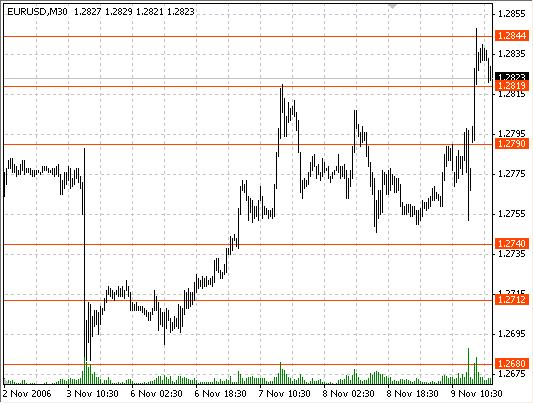The article also tackles the useful theme of creating a simple indicator that can display the results of different timeframes in one wrokspace. You can see an example below.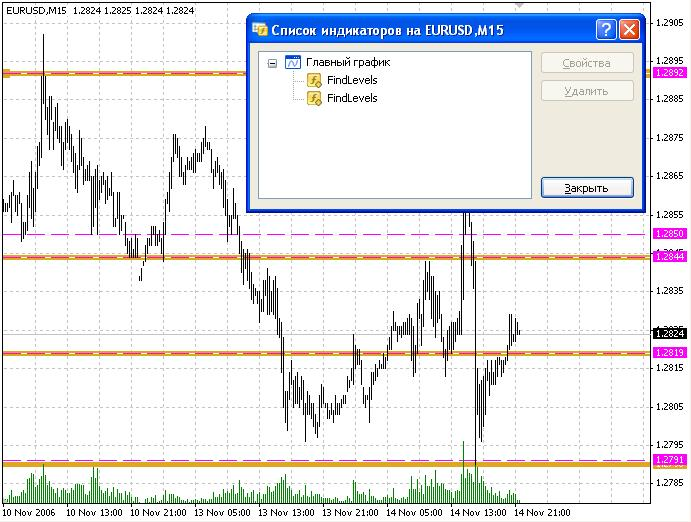The first indicator displays support levels (thick beige lines) based on 30-minute quotes. The second indicator running in the same window displays the levels based on 15-minute timeframe (thin dashed purple lines) above the 30-minute levels. See the “Indicators Interaction” section for more details.

This present article is a sequel of the article about creating a script for detecting support levels, but the difference between them is that this article has been written for those who find themselves at the advanced level in programming and using the MetaTrader 4 platform. That is why my recommendation to the beginners and all those who find this article too complicated is to start with my previous article named A Method of Drawing the Support/Resistance Levels.

Theoretical Review

Let us describe the algorithm for detecting support/resistance levels, which will be further realized in the FindLevels indicator. A support/resistance level is a price value that cannot be crossed due to some forces. It can be the forces induced by psychological mark, the influence of some big players or a big amount of StopLoss orders in this area. It is obvious that the quotes will cross this line far less frequently than the other lines which are not support ones. You can find the evidence of this fact in many trading books.

To take advantage of this fact we have to calculate the quantity of the bars that cross each price. This is a result of the calculations described in the previous article: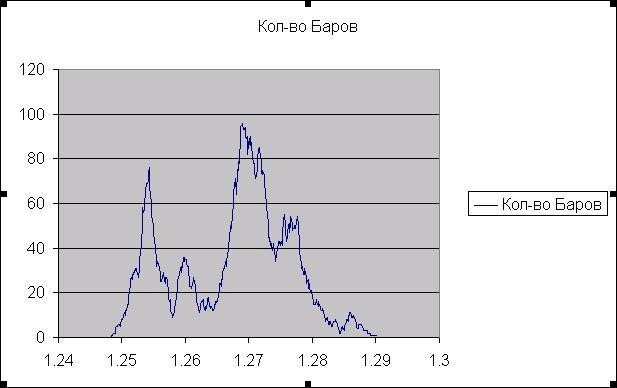The horizontal axis in this picture is a price; the vertical axis marks the quantity of the bars crossing the price. The chart has a lot of local minimums as you can see in the picture. A local minimum is a point that belongs to a non-zero interval and itself is the minimum of this interval. Now we have to select the local minimums according to a certain characteristic.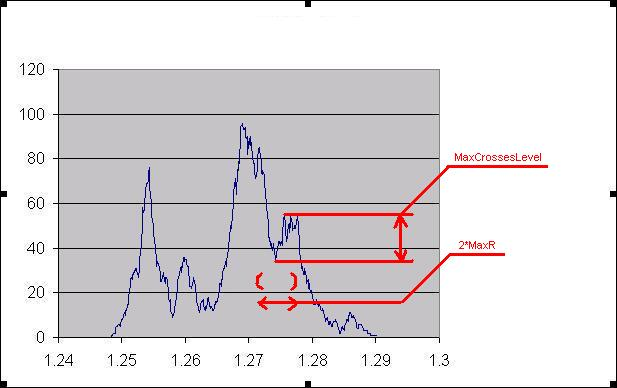First, we will set a constant, MaxR, which is the area radius. If a local minimum is not the minimum that belongs to the MaxR radius area, then it does not suit our task. Second, we will set MaxCrossesLevel parameter. If the maximum of function differs from the minimum less than by the MaxCrossesLevel in the MaxR area, we will not display this local minimum, as it is not significant enough. This is the mechanism of detecting support/resistance levels. Following this simple algorithm, we will write the indicator.

### Auxiliary Functions

As it was described above, the FindLevels indicator is designed for working with quotes from any timeframe. The timeframe is set by the user (TimePeriod variable). For the sake of simplicity of the code, we will set two easy functions that do not require additional explanations:

```double prLow(int i)
{
return (iLow(NULL,TimePeriod,i));
}

double prHigh(int i)
{
return (iHigh(NULL,TimePeriod,i));
}```

The third and the fourth functions are necessary for the convenient displaying of the support levels, their width, color and the indication mode that will depend on the timeframe:

```int Period2Int(int TmPeriod)
{
switch(TmPeriod)
{
case PERIOD_M1  : return(0);
case PERIOD_M5  : return(1);
case PERIOD_M15 : return(2);
case PERIOD_M30 : return(3);
case PERIOD_H1  : return(4);
case PERIOD_H4  : return(5);
case PERIOD_D1  : return(6);
case PERIOD_W1  : return(7);
case PERIOD_MN1 : return(8);
}
return (0);
}

string Period2AlpthabetString(int TmPeriod)
{
return(Alphabet[Period2Int(TmPeriod)]);
}```

The sense of setting the function Period2AlphabetString() is described in the “Indicators Interaction" section, and the use of Period2Int() function will be clear from the next section.

### Writing the Indicator

Let us start with the external variables, which are set by the user:

```extern int MaxLimit = 1000;
extern int MaxCrossesLevel = 10;
extern double MaxR = 0.001;
extern int TimePeriod = 0;
extern color LineColor = White;
extern int LineWidth = 0;
extern int LineStyle = 0;   ```
• MaxLimit - the amount of historical bars in use;
• MaxCrossesLevel – the minimal difference between the local maximum and the local minimum (see the detailed description in the "Theoretical Review” section;
• MaxR – the radius of the area where the minimum is detected;
• TimePeriod – the timeframe for detecting the support levels. By default, it is the timeframe of the mapping window;
• LineColor – the color of the displayed lines;
• LineWidth – the line width, 0 by default;
• LineStyle – the line style, 0 by default.

If the LineColor, LineWidth or LineStyle values are set by the user as by default, while performing the Init procedure we will change them into the other ones that will depend on the timeframe. Then the view of the lines from different timeframes will not be identical and we will be able differentiate them easily.

```int init()
{
if(TimePeriod == 0)
TimePeriod = Period();

if(TimePeriod != 0 && LineWidth == 0)
if(Period2Int(TimePeriod) - Period2Int(Period()) >= 0)
LineWidth = Widths[Period2Int(TimePeriod) - Period2Int(Period())];
else
{
LineWidth = 0;
if(LineStyle == 0)
LineStyle = STYLE_DASH;
}

if(TimePeriod != 0 && LineColor == White)
LineColor = Colors[Period2Int(TimePeriod)];

return(0);
}```

In the first line we set the TimePeriod value if it has been set by default. Then we determine the line width. The more the TimePeriod value in relation to the timeframe of the chart (mapping window) is, the wider are the lines. If the TimePeriod is less than the chart period, the line width is equal to 0 and the line is dashed. Each time period has its own color.

The arrays Colors[] and Width[] are defined in the following way:

```color  Colors[] = {Red,Maroon, Sienna, OrangeRed, Purple,I ndigo,
DarkViolet, MediumBlue, DarkSlateGray};
int    Widths[] = {1, 2, 3, 4, 5, 6, 7, 8, 9};```

Let us define the remaining variables:

```int CrossBarsNum[];
bool CrossBarsMin[];
double d1Num = 0.0, d2Num = 0.0;
datetime TMaxI = 0;```
• The array CrossBarsNum[] – the array indicating the amount of bars for each price;
• CrossBarsMin[] – the array corresponding to the fact whether the line with the set price is a local minimum or not;
• d1Num and d2Num - the minimum and the maximum prices which belong to the bar interval from 0 to MaxLimit;
• TMaxI – indicating the time of the last processed bar.
```#define MaxLines 1000
string LineName[MaxLines];
int LineIndex = 0;```
• MaxLines -the maximum amount of the lines which you will create;

• LineName[] – the array of their names;

• LineIndex – the index of the empty cell in the array LineName[].

Let us pass on the function start():

```int counted_bars = IndicatorCounted();
int limit = MathMin(Bars - counted_bars, MaxLimit);

double d1 = prLow(iLowest(NULL, TimePeriod, MODE_LOW, limit, 0));
double d2 = prHigh(iHighest(NULL, TimePeriod, MODE_HIGH, limit, 0));```

We calculate the limit variable using the quantity of the bars that has not been changed since the last fetch of the indicator. d1 and d2 are the minimum and the maximum of the price at the interval from 0 to limit.

```if(d1Num != d1 || d2Num != d2)
{
ArrayResize(CrossBarsNum, (d2 - d1)*10000);
ArrayResize(CrossBarsMin, (d2 - d1)*10000);

if(d1Num != d1 && d1Num != 0.0)
{
ArrayCopy(CrossBarsNum, CrossBarsNum, 0, (d1Num - d1)*10000);
ArrayCopy(CrossBarsMin, CrossBarsMin, 0, (d1Num - d1)*10000);
}

d1Num = d1;
d2Num = d2;
}```

During the indicator operation, the price gaps covered with the CrossBarsNum[] and CrossBarsMin[] arrays can change. Every time when it happens we have to increase the quantity of the cells in the array and to move it to the right if necessary. It usually happens if the new variables d1, d2 do not match the variables d1Num, d2Num which have been gotten at the previous launch of the function start().

```for(double d = d1; d <= d2; d += 0.0001)
{
int di = (d - d1)*10000;
for(int i = 1; i < limit; i++)
if(d > prLow(i) && d < prHigh(i))
CrossBarsNum[di]++;
if(Time[limit] != TMaxI&&TMaxI != 0)
if(d > prLow(iBarShift(NULL, 0, TMaxI)) &&
d < prHigh(iBarShift(NULL, 0, TMaxI)))
CrossBarsNum[di]--;
}
TMaxI = Time[limit] - 1;```

After we have made sure that our arrays fit the necessary dimensions, we start to calculate the new bars for each price and to increase the CrossBarsNum[] value when the bar crosses the price level. As the news bars are constantly emerging, the old bars will be excluded from the [0 : limit] interval. For this reason we have to check such kind of bars and to decrease the CrossBarsNum[] value in case of intersection. Then we will assign the time of the last calculated bar to the TmaxI variable.

```double l = MaxR*10000;
for(d = d1 + MaxR; d <= d2 - MaxR; d += 0.0001)
{
di = (d - d1)*10000;
if(!CrossBarsMin[di] && CrossBarsNum[ArrayMaximum(CrossBarsNum, 2*l, di - l)] -
CrossBarsNum[ArrayMinimum(CrossBarsNum, 2*l, di - l)] > MaxCrossesLevel &&
CrossBarsNum[di] == CrossBarsNum[ArrayMinimum(CrossBarsNum, 2*l, di - l)] &&
CrossBarsNum[di-1] != CrossBarsNum[ArrayMinimum(CrossBarsNum, 2*l, di - l)])
{
CrossBarsMin[di] = true;
LineName[LineIndex] = Period2AlpthabetString(TimePeriod) + TimePeriod + "_" + d;
ObjectCreate(LineName[LineIndex], OBJ_HLINE, 0, 0, d);
ObjectSet(LineName[LineIndex], OBJPROP_COLOR, LineColor);
ObjectSet(LineName[LineIndex], OBJPROP_WIDTH, LineWidth);
ObjectSet(LineName[LineIndex], OBJPROP_STYLE, LineStyle);
LineIndex++;
}
if(CrossBarsMin[di] && CrossBarsNum[di] !=
CrossBarsNum[ArrayMinimum(CrossBarsNum, 2*l, di - l)])
{
CrossBarsMin[di] = false;
ObjectDelete(Period2AlpthabetString(TimePeriod) + TimePeriod + "_" + d);
}
}```

At the end of the start() procedure we monitor the CrossBarsMin[] array once again in order to determine the new local minimums and to delete the old ones which turn out not to be the local minimums anymore. As it is more than one local minimum possible (there are several matching values in the CrossBarsMin[] array and they all are the local minimums), we have to derive only one of them. We will use the local minimum with the lowest price.

```CrossBarsNum[di] == CrossBarsNum[ArrayMinimum(CrossBarsNum, 2*l, di - l)] &&
CrossBarsNum[di-1]!= CrossBarsNum[ArrayMinimum(CrossBarsNum, 2*l, di - l)]```

There is nothing complicated in creating a new graphical object –horizontal line. We set the features for this line: the width, the style, the color, that have been designed previously in the Init procedure. There is nothing complicated in deleting the levels which turn out not to be support levels anymore. There is one thing that is not clear: why and for what purpose we use the Period2AlpthabetString(TimePeriod) function in the name of the object. The next paragraph describes this issue, which has been several times mentioned before.

### Indicators Interaction

As it was mentioned at the beginning of the article, the FindLevels indicator is designed for indicating the support levels of several timeframes in the one chart. In order to achieve this we need the following things:

1. It should be possible to launch the indicator several times and the indicator should have the input data with the time period;

2. The lines should be different and we should easily define what time period each support level belongs to;

3. All the lines should be traced both from long and short period of time.

The first point doesn’t cause any problems. We have no global variables. There are different names of graphical objects for each time period, as we have the period in the name of the object, (e.g. “f30_1.25600000”, 30 is the time period), and so there will be no conflicts when we launch the several indicators.

The second point is realized successfully, as each line has its color depending on the period (LineColor=Colors[Period2Int(TimePeriod)]).

Now we have the third point left. It is quite logical that if a line turns out to be the support line for 5-minutes chart, it will be also the support line for 30-minutes chart. If these lines clash in price and have the same width, we will just not see one of the lines! That is why the lines of different timeframes should differ in width. We will make the support levels of the longer timeframes wider than the lines of the shorter ones. It is quite reasonable, because the lines belonging to the long intervals are more significant.

We have to set the proper priority of displaying the lines. The thinner lines should be displayed at the end and should overlay the thick lines, so that they can be seen clearly. In the MetaTrader 4 program the objects are displayed in alphabetical order. So it is necessary that the names of the lines of the longer periods should alphabetically come prior to the lines of the shorter periods. That is why we have created the function which sets the Latin alphabet letter depending on the period:

```string Period2AlpthabetString(int TmPeriod)
{
return (Alphabet[Period2Int(TmPeriod)]);
}```

Alphabet is the array of the Latin letters in reverse order. The full name of each support level is the following: Period2AlpthabetString(TimePeriod)+TimePeriod+"_"+d.

For better understanding let me refer to the screenshot from the beginning of the article:### Summary

The testing of the indicator showed that it works fine. It is easy to use because it can display the data from the different timeframes. The testing period showed that it is more convenient if the indicator displays 3-10 support levels for each TimePeriod. To achieve this, we have to select the corresponding input entries MaxR and MaxCrossesLevel. During the testing MaxR varied from 0.0003 for shorter timeframes to 0.002 for longer ones. MaxCrossesLevel varied from 3 to 20. Perhaps it would be helpful to configure the indicator so that it could display the certain amount of the most significant support levels, but if so, it would have made the code more complicated. I think those who like my indicator can easily do it themselves.

Translated from Russian by MetaQuotes Software Corp.
Original article: https://www.mql5.com/ru/articles/1440

Attached files |
FindLevels.mq4 (5.21 KB)

#### Other articles by this author

Last comments | Go to discussion (8)| 23 May 2011 at 23:06
I have analyzed the code and found problems. In no particular order:
1. ```#define MaxLines 1000
string LineName[MaxLines];
int LineIndex = 0;
...
ObjectSet(LineName[LineIndex], OBJPROP_STYLE, LineStyle);
LineIndex++;
```
LineIndex and LineName[] is never adjusted when lines are moved.
2. ```int counted_bars = IndicatorCounted();
int limit = MathMin(Bars - counted_bars, MaxLimit);

double d1 = prLow(iLowest(NULL, TimePeriod, MODE_LOW, limit, 0));
double d2 = prHigh(iHighest(NULL, TimePeriod, MODE_HIGH, limit, 0));
```
Except for the first pass, limit will be zero and d1,d2 garbage, and the rest of the algorithm fails.
3. ```if(d1Num != d1 && d1Num != 0.0){
ArrayCopy(CrossBarsNum, CrossBarsNum, 0, (d1Num - d1)*10000);
```
The code assumes 4 digits, would be extremely slow on they Yen. I don't think that the copy moves counts in the correct direction (up or down is possible. )
4. ``` if(Time[limit] != TMaxI&&TMaxI != 0)
if(d > prLow(iBarShift(NULL, 0, TMaxI)) &&
d < prHigh(iBarShift(NULL, 0, TMaxI)))
CrossBarsNum[di]--;
}
TMaxI = Time[limit] - 1;```
Definitely should be Time[limit-1] but still has other problems such as moving multiple bars from counts.
5. ```ArrayResize(CrossBarsNum, (d2 - d1)*10000);
```
Array size one pip too small, need d1 to d2 inclusive.
6. ```di = (d - d1)*10000;
if(!CrossBarsMin[di] && CrossBarsNum[ArrayMaximum(CrossBarsNum, 2*l, di - l)]
```
ArrayMaximum scans beyond array bounds

So I rewrote the code but kept the same functionality.
The original code took about ten seconds to refresh. My code is instantaneous.| 26 May 2011 at 22:16
I didn't like the MaxCrossesLevel and MaxR idea. For one it doesn't show the min/max lines because of long tails. I replaced that portion with different algorithm.
1. Roll down hill and find the lowest low. Line found.
2. Climb uphill after the lowest low.
3. Roll down hill forward from the last highest high.
4. ...[Deleted] | 7 May 2013 at 09:56
WHRoeder:
I have analyzed the code and found problems. In no particular order:
1. ```#define MaxLines 1000
string LineName[MaxLines];
int LineIndex = 0;
...
ObjectSet(LineName[LineIndex], OBJPROP_STYLE, LineStyle);
LineIndex++;
```
LineIndex and LineName[] is never adjusted when lines are moved.
2. ```int counted_bars = IndicatorCounted();
int limit = MathMin(Bars - counted_bars, MaxLimit);

double d1 = prLow(iLowest(NULL, TimePeriod, MODE_LOW, limit, 0));
double d2 = prHigh(iHighest(NULL, TimePeriod, MODE_HIGH, limit, 0));
```
Except for the first pass, limit will be zero and d1,d2 garbage, and the rest of the algorithm fails.
3. ```if(d1Num != d1 && d1Num != 0.0){
ArrayCopy(CrossBarsNum, CrossBarsNum, 0, (d1Num - d1)*10000);
```
The code assumes 4 digits, would be extremely slow on they Yen. I don't think that the copy moves counts in the correct direction (up or down is possible. )
4. ``` if(Time[limit] != TMaxI&&TMaxI != 0)
if(d > prLow(iBarShift(NULL, 0, TMaxI)) &&
d < prHigh(iBarShift(NULL, 0, TMaxI)))
CrossBarsNum[di]--;
}
TMaxI = Time[limit] - 1;```
Definitely should be Time[limit-1] but still has other problems such as moving multiple bars from counts.
5. ```ArrayResize(CrossBarsNum, (d2 - d1)*10000);
```
Array size one pip too small, need d1 to d2 inclusive.
6. ```di = (d - d1)*10000;
if(!CrossBarsMin[di] && CrossBarsNum[ArrayMaximum(CrossBarsNum, 2*l, di - l)]
```
ArrayMaximum scans beyond array bounds

So I rewrote the code but kept the same functionality.
The original code took about ten seconds to refresh. My code is instantaneous.

Hi,  Have you tried to include the Fractal in the algoritm?. It would increase a filtering of low/high from the  indicator and let more accurated S/R levels.
WHRoeder:
I didn't like the MaxCrossesLevel and MaxR idea. For one it doesn't show the min/max lines because of long tails. I replaced that portion with different algorithm.
1. Roll down hill and find the lowest low. Line found.
2. Climb uphill after the lowest low.
3. Roll down hill forward from the last highest high.
4. ...| 22 Jan 2019 at 21:53
whroeder1 2011.05.26 16:16: I have analyzed the code and found problems. ... So I rewrote the code but kept the same functionality.

Code was lost over the last 8 years. I've attached what I believe was the modified file.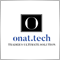| 10 May 2020 at 07:20
William Roeder:

Code was lost over the last 8 years. I've attached what I believe was the modified file.

Good morning from Turkey. Good morning from Turkey. Thank yo very much for this usefull code.

The attached file works fine, except the lower horizontal line dissapers and not seen again after the backtest starts. I checked the code several times, still I didnt get why it happens. I gave my own inputs; 100,1,0.01,Yellow,0,3,5. Do I miss something about this code? Or is there something really wrong  and I cant catch it?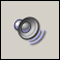Sound Alerts in Indicators
How to create "voice" indicators for daily usage.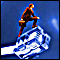A Method of Drawing the Support/Resistance Levels
This article describes the process of creating a simple script for detecting the support/resistance levels. It is written for beginners, so you can find the detailed explanation of every stage of the process. However, though the script is very simple, the article will be also useful for advanced traders and the users of the MetaTrader 4 platform. It contains the examples of the data export into the tabular format, the import of the table to Microsoft Excel and plotting the charts for the further detailed analysis.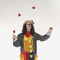Simultaneous Displaying of the Signals of Several Indicators from the Four Timeframes
While manual trading you have to keep an eye on the values of several indicators. It is a little bit different from mechanical trading. If you have two or three indicators and you have chosen a one timeframe for trading, it is not a complicated task. But what will you do if you have five or six indicators and your trading strategy requires considering the signals on the several timeframes?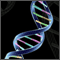Genetic Algorithms vs. Simple Search in the MetaTrader 4 Optimizer
The article compares the time and results of Expert Advisors' optimization using genetic algorithms and those obtained by simple search.